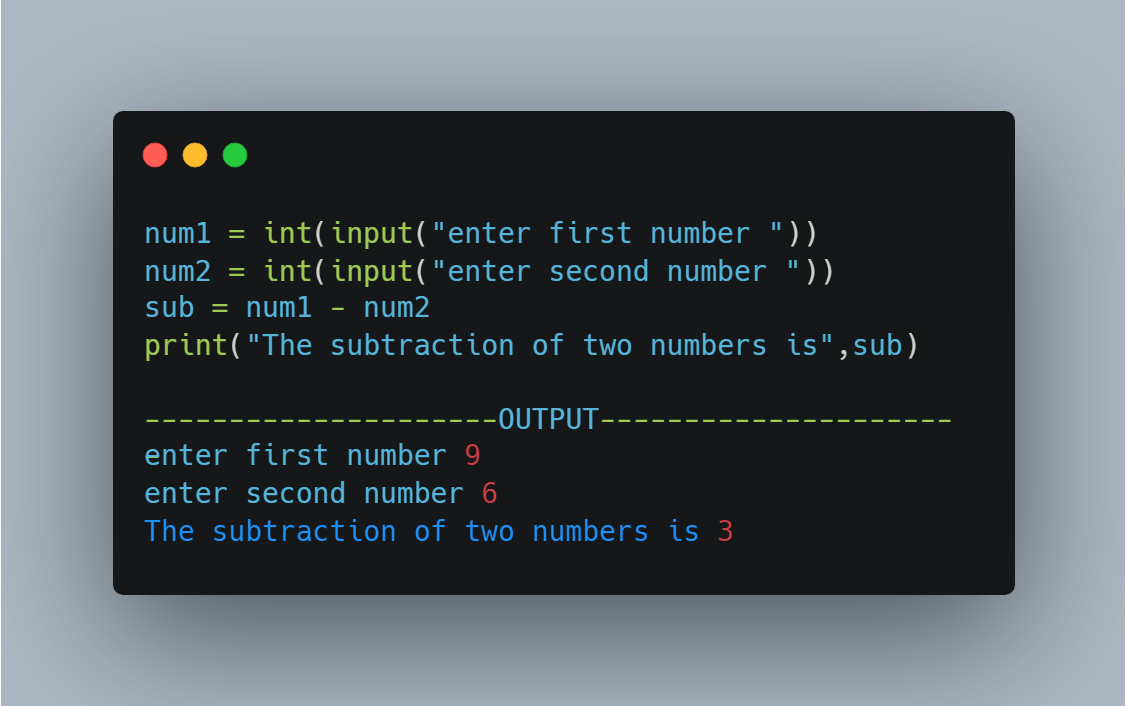# Making Simple Calculator

After understanding the use of input and print statements, next, we are going to learn about making a simple calculator. Here we will perform all sorts of calculations one by one and understand them. In the end, we will make a calculator which will do all the calculations in a single program.

Here we will take input from the user and then print the addition of two numbers.

So in the above code, we took input from the user and then store the values entered by the user in a separate variable, and then we did the addition of both the numbers.

The next thing we will do is subtract two numbers.

## Subtracting Two Numbers

We will do the same process we did before in adding two numbers.Taking input from the user and subtracting the numbers to get the result

So the subtraction of two numbers was easy to do.

## Multiplying Two Numbers

We will take input from the user and then multiply them to get the output.

The multiplication of two numbers is also simple.

The next step is for dividing two numbers.

## Dividing Two Numbers

Division of Numbers can be done in two ways:-

1. Float Division(/)
2. Integer Division(//)

First, we will discuss the Float Division, this method will give the output in decimal form and it is the basic and most commonly used operator from which we do division.

So in the above code, we did a division of two numbers and we can clearly see from the output that it is in decimal form.

Now moving on to Integer Division

Here we will get the input in Integer form

It is clearly visible from the above code that the output is in Integer form.

## Modulus of Two Numbers

Now the last step we will learn here is of finding the modulus of two numbers.

We use modulus to get the remainder of the division.

## Creating Calculator

So finally we had made a calculator that can perform all simple and basic calculations.

For more reference, refer to this video

If you are interested in learning from videos then subscribe to this channel on youtube.

For python related quizzes, posts, and memes you can follow us on Instagram and also on Twitter.

Happy Learning. Thanks for reading. Stay tuned and subscribe to our newsletter. Many more to come shortly!!!

NOTE:- The awesome artwork used in this article was created by Dmytro Novitskyi